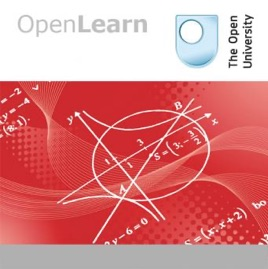1 episódio

Attempts to answer problems in areas as diverse as science, technology and economics involve solving simultaneous linear equations. In this unit we look at some of the equations that represent points, lines and planes in mathematics. We explore concepts such as Euclidean space, vectors, dot products and conics. This study unit is just one of many that can be found on LearningSpace, part of OpenLearn, a collection of open educational resources from The Open University. Published in ePub 2.0.1 format, some feature such as audio, video and linked PDF are not supported by all ePub readers.# Vectors and conics - for iBooks The Open University

• Educação

Attempts to answer problems in areas as diverse as science, technology and economics involve solving simultaneous linear equations. In this unit we look at some of the equations that represent points, lines and planes in mathematics. We explore concepts such as Euclidean space, vectors, dot products and conics. This study unit is just one of many that can be found on LearningSpace, part of OpenLearn, a collection of open educational resources from The Open University. Published in ePub 2.0.1 format, some feature such as audio, video and linked PDF are not supported by all ePub readers.